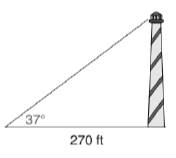Chapter 11.3, Problem 38E### Elementary Geometry for College St...

6th Edition
Daniel C. Alexander + 1 other
ISBN: 9781285195698

#### Solutions

Chapter
Section### Elementary Geometry for College St...

6th Edition
Daniel C. Alexander + 1 other
ISBN: 9781285195698
Textbook Problem
1 views

# In Exercises 37 to 43, angle measures should be given to the nearest degree; distance should be given to the nearest tenth of a unit.Kristine observes the top of a lookout tower from a point 270 ft from its base. If the indicated angle of elevation measures 37 ∘ , how tall is the tower?To determine

To calculate:

The height of the tower.

Explanation

Given:

Kristine observes the top of a lookout tower from a point 270 ft from its base. The angle of elevation is 37°.

Definition used:

The trigonometric ratio

Calculation:

Consider the angle as α.

Thus α=37.

We need to find the height of the tower, that is, the length of the opposite leg.

Let the height of the tower be x.

That is, the length of the opposite leg is x.

From the figure, we see that tanα=x270.

tan37=x270

x=270tan37

Let us use Ti-83 calculator to find the value of x

### Still sussing out bartleby?

Check out a sample textbook solution.

See a sample solution

#### The Solution to Your Study Problems

Bartleby provides explanations to thousands of textbook problems written by our experts, many with advanced degrees!

Get Started

#### Find more solutions based on key concepts